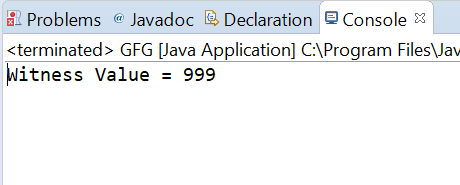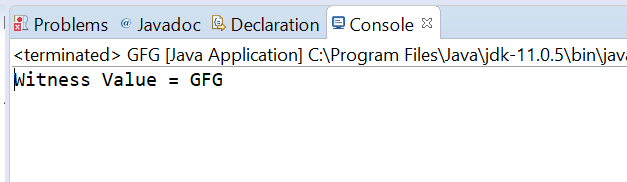Related Articles
AtomicReference compareAndExchange() method in Java with Examples
• Last Updated : 27 Dec, 2019

The compareAndExchange() method of a AtomicReference class is used to Atomically sets the value to newValue to AtomicReference object, if the current value of AtomicReference object which is referred to as the witness value is equal to the expectedValue.This method will return the witness value, which will be the same as the expected value. This method handles the operation with memory semantics of reading as if the variable was declared volatile.

Syntax:

```public final V compareAndExchange(V expectedValue,
V newValue)
```

Parameters: This method accepts expectedValue which is the expected value and newValue which is the new value to set.

Return value: This method returns the witness value, which will be the same as the expected value if successful.

Below programs illustrate the compareAndExchange() method:
Program 1:

 `// Java program to demonstrate``// AtomicReference.compareAndExchange() method`` ` `import` `java.util.concurrent.atomic.AtomicReference;`` ` `public` `class` `GFG {``    ``public` `static` `void` `main(String[] args)``    ``{`` ` `        ``// create an atomic reference object``        ``// which stores Integer.``        ``AtomicReference ref``            ``= ``new` `AtomicReference();`` ` `        ``// set some value``        ``ref.set(``999``);`` ` `        ``// apply compareAndExchange()``        ``Integer oldValue``            ``= ref.compareAndExchange(``                ``999``,``                ``999999``);`` ` `        ``// print value``        ``System.out.println(``"Witness Value= "``                           ``+ oldValue);``    ``}``}`
Output:Program 2:

 `// Java program to demonstrate``// AtomicReference.compareAndExchange() method`` ` `import` `java.util.concurrent.atomic.AtomicReference;`` ` `public` `class` `GFG {``    ``public` `static` `void` `main(String[] args)``    ``{`` ` `        ``// create an atomic reference object.``        ``AtomicReference ref``            ``// = new AtomicReference();`` ` `            ``// set some value``            ``ref.set(``"GFG"``);`` ` `        ``// apply compareAndExchange()``        ``String oldValue``            ``= ref.compareAndExchange(``                ``"GFG"``,``                ``"Geeks for Geeks"``);`` ` `        ``// print value``        ``System.out.println(``"Witness Value= "``                           ``+ oldValue);``    ``}``}`
Output:Attention reader! Don’t stop learning now. Get hold of all the important Java Foundation and Collections concepts with the Fundamentals of Java and Java Collections Course at a student-friendly price and become industry ready. To complete your preparation from learning a language to DS Algo and many more,  please refer Complete Interview Preparation Course.

My Personal Notes arrow_drop_up